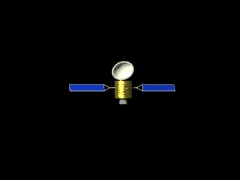Ch 8. Rigid Body Momentum/Impulse Multimedia Engineering Dynamics Impulse Momentum Eccentric Impact
 Chapter - Particle - 1. General Motion 2. Force & Accel. 3. Energy 4. Momentum - Rigid Body - 5. General Motion 6. Force & Accel. 7. Energy 8. Momentum 9. 3-D Motion 10. Vibrations Appendix Basic Math Units Basic Equations Sections Search eBooks Dynamics Fluids Math Mechanics Statics Thermodynamics Author(s): Kurt Gramoll ©Kurt GramollDYNAMICS - CASE STUDY IntroductionProblem Diagram A communications satellite is struck by a meteoroid and sent spinning. Half a second later, the satellite's thrusters fire opposite the rotation to stop the spinning. What is known: meteoroid momentum pm = 0.33 kg-km/s impact angle α = 45° satellite mass ms = 100 kg satellite moment of inertia Is = 150 kg-m2 thruster force F = 200 N each initial angular velocity is zero before impact QuestionProblem Graphic How long must the thrusters fire in order to stop the satellite from spinning? Approach Assume the meteoroid becomes imbedded in the satellite. Using the Conservation of Momentum, determine the motion of the satellite after the impact. Using the Principle of Impulse and Momentum, determine the thruster firing-time required to reduce the rotational velocity back to zero.

Practice Homework and Test problems now available in the 'Eng Dynamics' mobile app
Includes over 400 problems with complete detailed solutions.
Available now at the Google Play Store and Apple App Store.